This is a simple analysis that I've done using R with Students Performance in Exams in Kaggle.

library(ggplot2)
library(dplyr)
library(gridExtra)
library(fastDummies)
library(caTools)
library(randomForest)
library(caret)
library(broom)

data <- read.csv('./data/StudentsPerformance.csv')
data <- data %>%
mutate(average = (reading.score + math.score + writing.score) / 3) %>%
mutate(race.ethnicity = substr(race.ethnicity, 7, 7)) %>%
mutate(test.preparation.course = test.preparation.course == "completed") %>%
mutate(lunch = lunch == "standard")
head(data)
# Edit the levels of education
levels(data$parental.level.of.education)[match("high school",levels(data$parental.level.of.education))] <- "hs"

levels(data$parental.level.of.education)[match("master's degree",levels(data$parental.level.of.education))] <- "md"

levels(data$parental.level.of.education)[match("bachelor's degree",levels(data$parental.level.of.education))] <- "bd"

levels(data$parental.level.of.education)[match("some college",levels(data$parental.level.of.education))] <- "c"

levels(data$parental.level.of.education)[match("associate's degree",levels(data$parental.level.of.education))] <- "as"

levels(data$parental.level.of.education)[match("some high school",levels(data$parental.level.of.education))] <- "h"

## Exploring the factor columns

explore_boxplot <- function(mv){
p <- list()
for (rv in names(data[, 6:9])) {
tmp <- ggplot(data) +
geom_boxplot(aes_string(x = mv, y = rv))
p[[length(p) + 1]] <- tmp
}
do.call(grid.arrange, c(p))
}

### Gender

explore_boxplot("gender")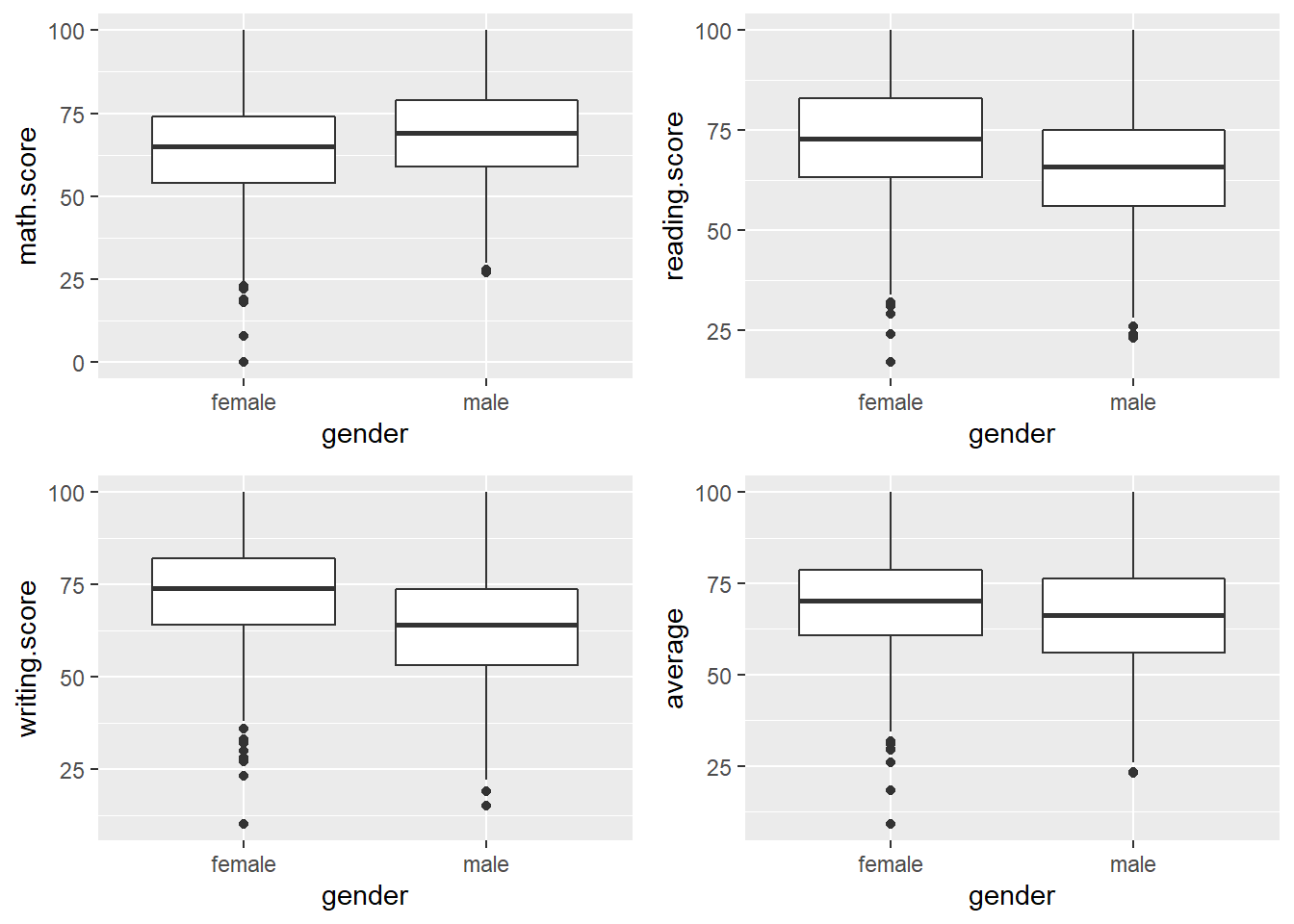• Looks like gender does affect the scores, on average both are quite balanced
• But males do better in Math while Females do better in writing & reading

### Race

explore_boxplot("race.ethnicity")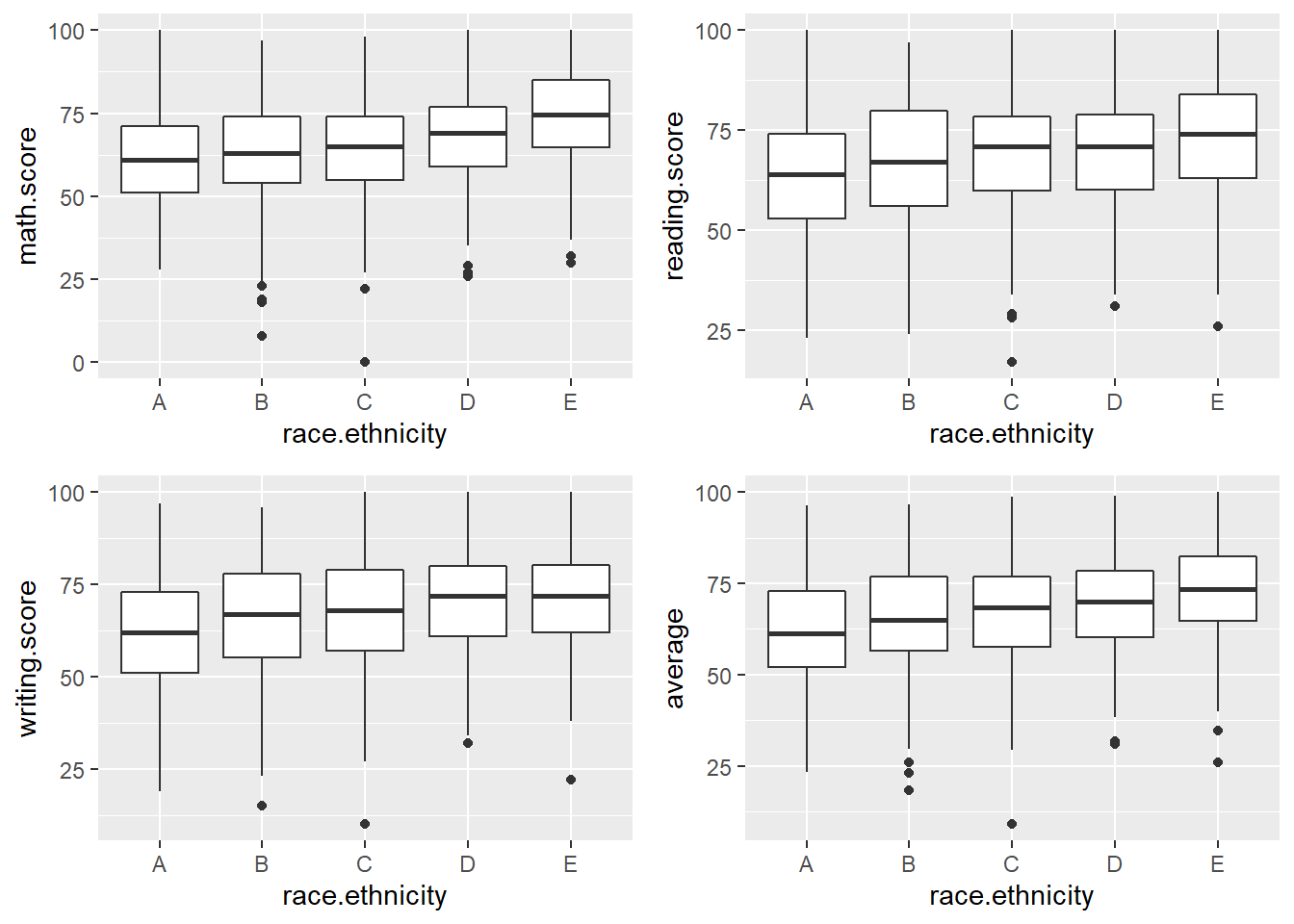• Can’t say for sure, but a rough look tells me that race will affect too!
• Race group E seems like doing better than other races while A looks like it is not doing as well.

### Lunch

explore_boxplot("lunch")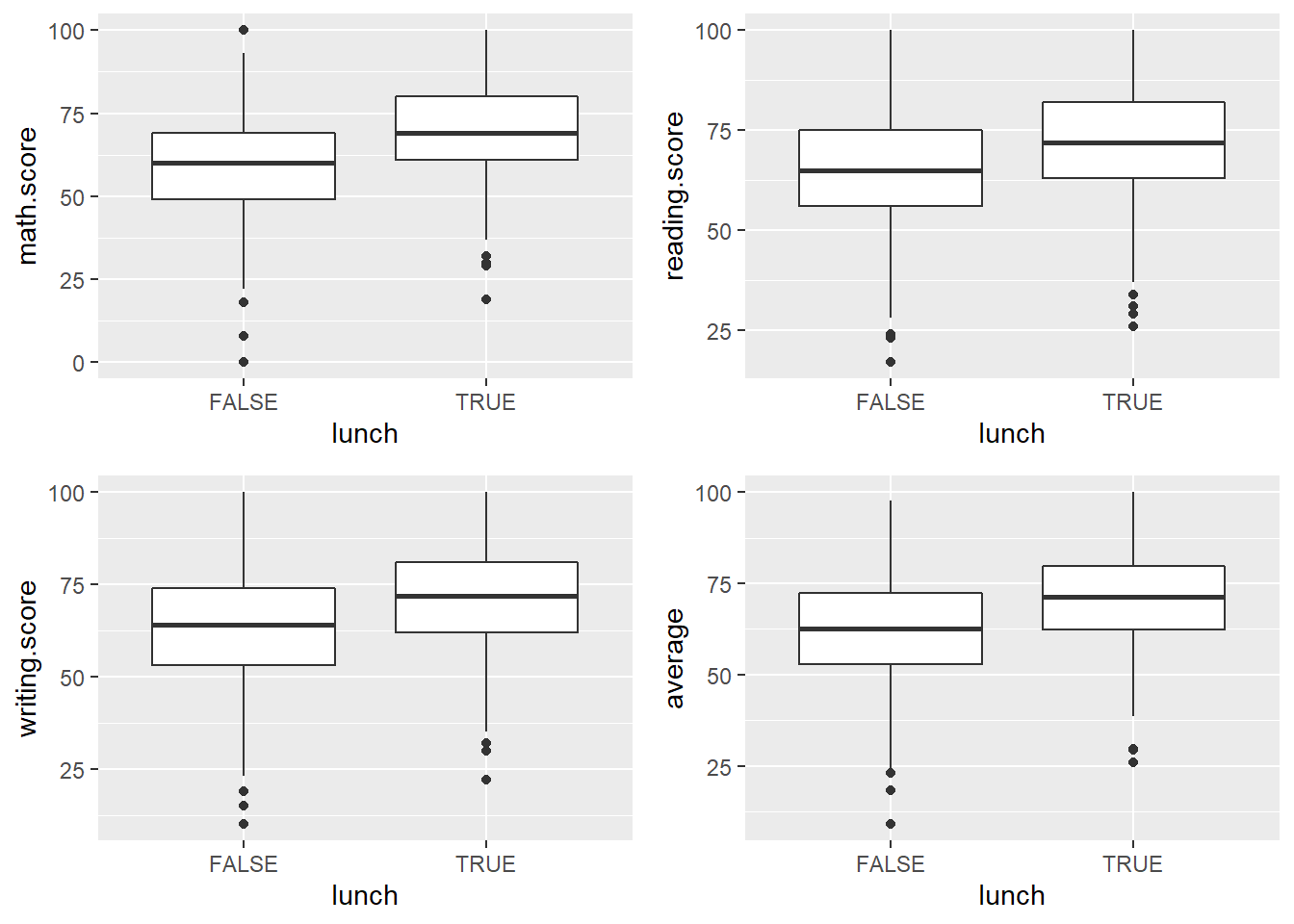• TRUE lunch indicates standard lunch
• This conforms to expectation where good lunch gives better score,
• So here can give a short conclusion: Eat good lunch for better score :D

### Test Preparation

explore_boxplot("test.preparation.course")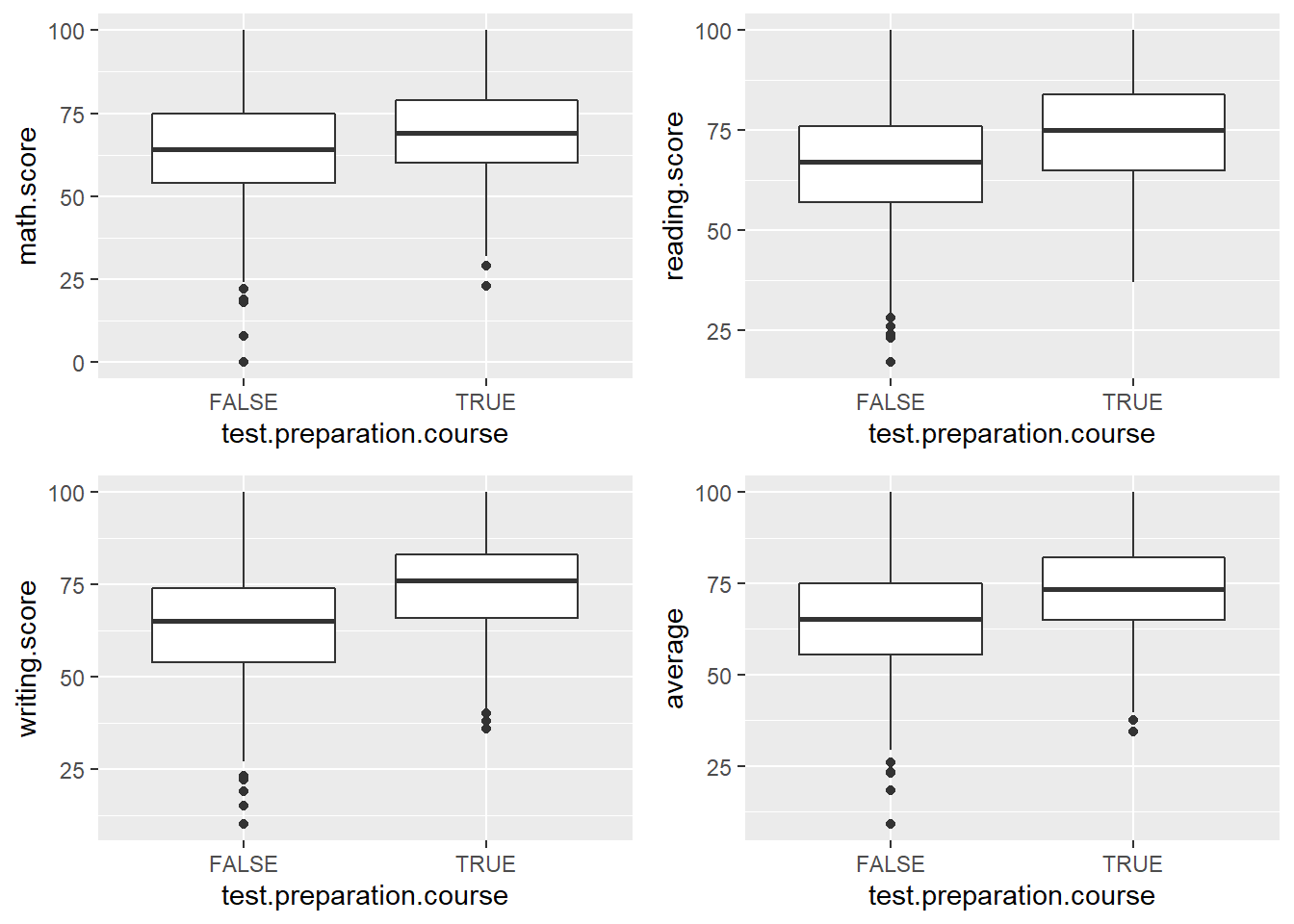• Again, as expected, the students who took test preparation course performed better than those who did not take.

### Parent's Education

explore_boxplot("parental.level.of.education")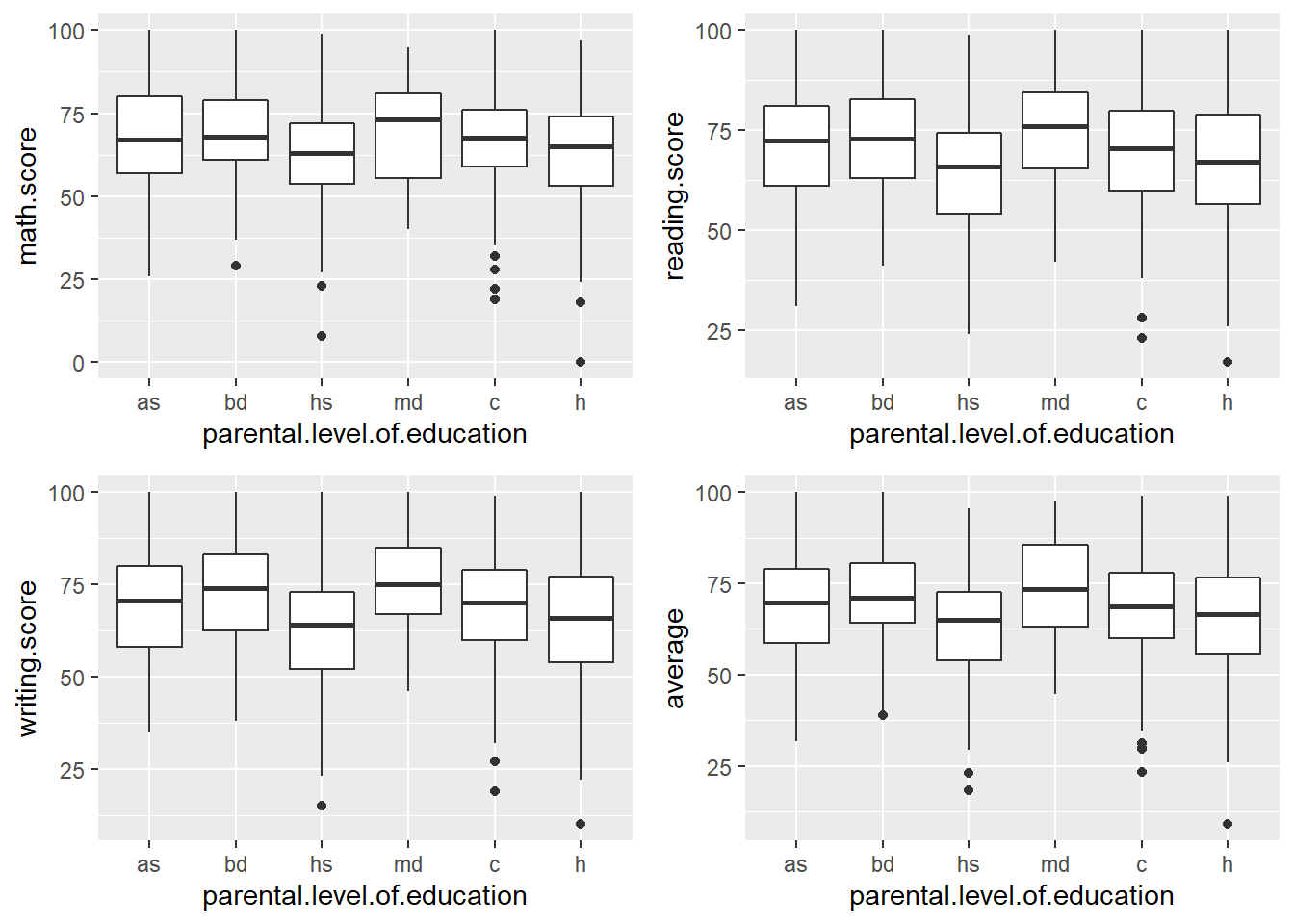• There definitely exists a relationship between parent’s education level and the children’s scores, but it isn’t as clear and direct as other relationships.
• A rough glance, though, tells me that students’ parents with just high school levelof education perform the worse.

## Confirming the relationship

• Unlike the other 4 properties, parent’s level of education’s effect on the student might not be as significant. Perhaps I will try a T test on a linear model with parent’s level of education.
• Assuming sigma = 0.05.
mod <- lm(average ~ parental.level.of.education, data = data)
summary(mod)
##
## Call:
## lm(formula = average ~ parental.level.of.education, data = data)
##
## Residuals:
##     Min      1Q  Median      3Q     Max
## -56.108  -9.259   0.874   9.895  33.892
##
## Coefficients:
##                               Estimate Std. Error t value Pr(>|t|)
## (Intercept)                    69.5691     0.9344  74.457  < 2e-16 ***
## parental.level.of.educationbd   2.3547     1.5860   1.485  0.13796
## parental.level.of.educationhs  -6.4721     1.3645  -4.743 2.41e-06 ***
## parental.level.of.educationmd   4.0298     2.0391   1.976  0.04840 *
## parental.level.of.educationc   -1.0927     1.3155  -0.831  0.40640
## parental.level.of.educationh   -4.4611     1.3985  -3.190  0.00147 **
## ---
## Signif. codes:  0 '***' 0.001 '**' 0.01 '*' 0.05 '.' 0.1 ' ' 1
##
## Residual standard error: 13.92 on 994 degrees of freedom
## Multiple R-squared:  0.05131,    Adjusted R-squared:  0.04654
## F-statistic: 10.75 on 5 and 994 DF,  p-value: 4.381e-10
• By using the sigma, we can see that parent’s high school level of education has a significant effect on the student while other levels not so significant.
• This conforms to the hypothesis I made earlier.

## Testing with a simple linear model

model <- lm(math.score ~ parental.level.of.education + test.preparation.course + lunch + gender + race.ethnicity, data = data)
summary(model)
##
## Call:
## lm(formula = math.score ~ parental.level.of.education + test.preparation.course +
##     lunch + gender + race.ethnicity, data = data)
##
## Residuals:
##     Min      1Q  Median      3Q     Max
## -50.357  -8.744   0.166   9.001  30.655
##
## Coefficients:
##                               Estimate Std. Error t value Pr(>|t|)
## (Intercept)                    52.1358     1.8115  28.780  < 2e-16 ***
## parental.level.of.educationbd   1.9661     1.5020   1.309 0.190831
## parental.level.of.educationhs  -4.8027     1.2971  -3.703 0.000225 ***
## parental.level.of.educationmd   2.8884     1.9382   1.490 0.136490
## parental.level.of.educationc   -0.5827     1.2470  -0.467 0.640431
## parental.level.of.educationh   -4.2487     1.3331  -3.187 0.001482 **
## test.preparation.courseTRUE     5.4947     0.8756   6.275 5.22e-10 ***
## lunchTRUE                      10.8768     0.8727  12.463  < 2e-16 ***
## gendermale                      4.9953     0.8390   5.954 3.63e-09 ***
## race.ethnicityB                 2.0408     1.6998   1.201 0.230181
## race.ethnicityC                 2.4700     1.5918   1.552 0.121060
## race.ethnicityD                 5.3410     1.6241   3.289 0.001042 **
## race.ethnicityE                10.1347     1.8015   5.626 2.41e-08 ***
## ---
## Signif. codes:  0 '***' 0.001 '**' 0.01 '*' 0.05 '.' 0.1 ' ' 1
##
## Residual standard error: 13.17 on 987 degrees of freedom
## Multiple R-squared:  0.2548, Adjusted R-squared:  0.2457
## F-statistic: 28.12 on 12 and 987 DF,  p-value: < 2.2e-16
• Looking at how some values are insignificant, perhaps I will create a dummy variable & split the data into training and test data.

## Creating dummy variables & cleaning the data

cleaned <- dummy_cols(data, select_columns = c("gender", "race.ethnicity",
"parental.level.of.education",
"lunch", "test.preparation.course"), remove_selected_columns = TRUE)

head(cleaned)
# Dropping redundant columns
redundants <- c("race.ethnicity_E", "gender_male", "parental.level.of.education_h", "lunch_TRUE", "test.preparation.course_TRUE")
cleaned <- cleaned[, !(names(cleaned) %in% redundants)]
head(cleaned)
splitted <- sample.split(cleaned$average, SplitRatio = 0.8) train <- cleaned[splitted == TRUE, ] test <- cleaned[splitted == FALSE, ] dim(train) ##  811 16 dim(test) ##  189 16 ## Various Models I am going to try some of the regression algorithm I am familiar with: 1. Linear Regression 2. Random Forest 3. KNN ### Linear Regression # A function that builds the formula based on variables given build_linear_formula <- function(label, variables) { f <- as.formula(paste(label, "~", paste(variables, collapse = "+"))) return(f) } #### Building the model linear_model <- lm(build_linear_formula("math.score", names(cleaned)[5:16]), data = train) summary(linear_model) ## ## Call: ## lm(formula = build_linear_formula("math.score", names(cleaned)[5:16]), ## data = train) ## ## Residuals: ## Min 1Q Median 3Q Max ## -49.257 -8.984 0.465 9.593 31.186 ## ## Coefficients: ## Estimate Std. Error t value Pr(>|t|) ## (Intercept) 78.8385 1.7978 43.854 < 2e-16 *** ## gender_female -5.1019 0.9391 -5.433 7.36e-08 *** ## race.ethnicity_A -9.6317 1.9874 -4.846 1.51e-06 *** ## race.ethnicity_B -7.4053 1.6811 -4.405 1.20e-05 *** ## race.ethnicity_C -6.2709 1.5260 -4.109 4.38e-05 *** ## race.ethnicity_D -3.9705 1.5595 -2.546 0.011085 * ## parental.level.of.education_as 4.9676 1.4891 3.336 0.000890 *** ## parental.level.of.education_bd 6.7571 1.7601 3.839 0.000133 *** ## parental.level.of.education_hs -0.3117 1.5396 -0.202 0.839609 ## parental.level.of.education_md 9.1215 2.1580 4.227 2.64e-05 *** ## parental.level.of.education_c 3.5386 1.4877 2.379 0.017615 * ## lunch_FALSE -11.7172 0.9756 -12.010 < 2e-16 *** ## test.preparation.course_FALSE -6.4910 0.9866 -6.579 8.57e-11 *** ## --- ## Signif. codes: 0 '***' 0.001 '**' 0.01 '*' 0.05 '.' 0.1 ' ' 1 ## ## Residual standard error: 13.28 on 798 degrees of freedom ## Multiple R-squared: 0.2757, Adjusted R-squared: 0.2648 ## F-statistic: 25.31 on 12 and 798 DF, p-value: < 2.2e-16 • This model doesn’t perform really well, as can be seen that R^2 is quite small, so not much of the variance can be explained by the model. #### Evaluating the model predict_linear <- predict(linear_model, test) linear_test <- test %>% mutate(test = predict_linear) %>% mutate(se = (test - math.score) ** 2) rmse_linear <- mean(linear_test$se) ** 0.5
rmse_linear
##  12.90526
• For this simple linear regression model, RMSE is about 12, will other algorithms do better?

### Random Forest

#### Training the model

rf_model <- randomForest(
build_linear_formula("math.score", names(cleaned)[5:16]),
train
)

#### Evaluating Random forest

predict_rf <- predict(rf_model, test)

rf_test <- test %>%
mutate(test = predict_rf) %>%
mutate(se = (test - math.score) ** 2)

rmse_rf <- mean(rf_test$se) ** 0.5 rmse_rf ##  13.71061 • Hmmm, looks like random forest performs about the same level as the linear regression. ### KNN #### Training the model knn_model <- knnreg( train[, 5:16], train[, 1] ) #### Evaluating KNN predict_knn <- predict(knn_model, test[, 5:16]) knn_test <- test %>% mutate(test = predict_knn) %>% mutate(se = (test - math.score) ** 2) rmse_knn <- mean(knn_test$se) ** 0.5
rmse_knn
##  14.01039

#### Trying different k values

my_knn <- function(n) {
knn_model_inner <- knnreg(
train[, 5:16],
train[, 1],
k = n)
predict_knn_inner <- predict(knn_model_inner, test[, 5:16])

knn_test_inner <- test %>%
mutate(test = predict_knn_inner) %>%
mutate(se = (test - math.score) ** 2)

rmse_knn <- mean(knn_test_inner$se) ** 0.5 return(rmse_knn) } res <- sapply(3:50, my_knn) nums <- 3:50 knn_multiple <- data.frame(n = nums) knn_multiple[["result"]] <- res ggplot(knn_multiple, aes(x = n, y = result)) + geom_line()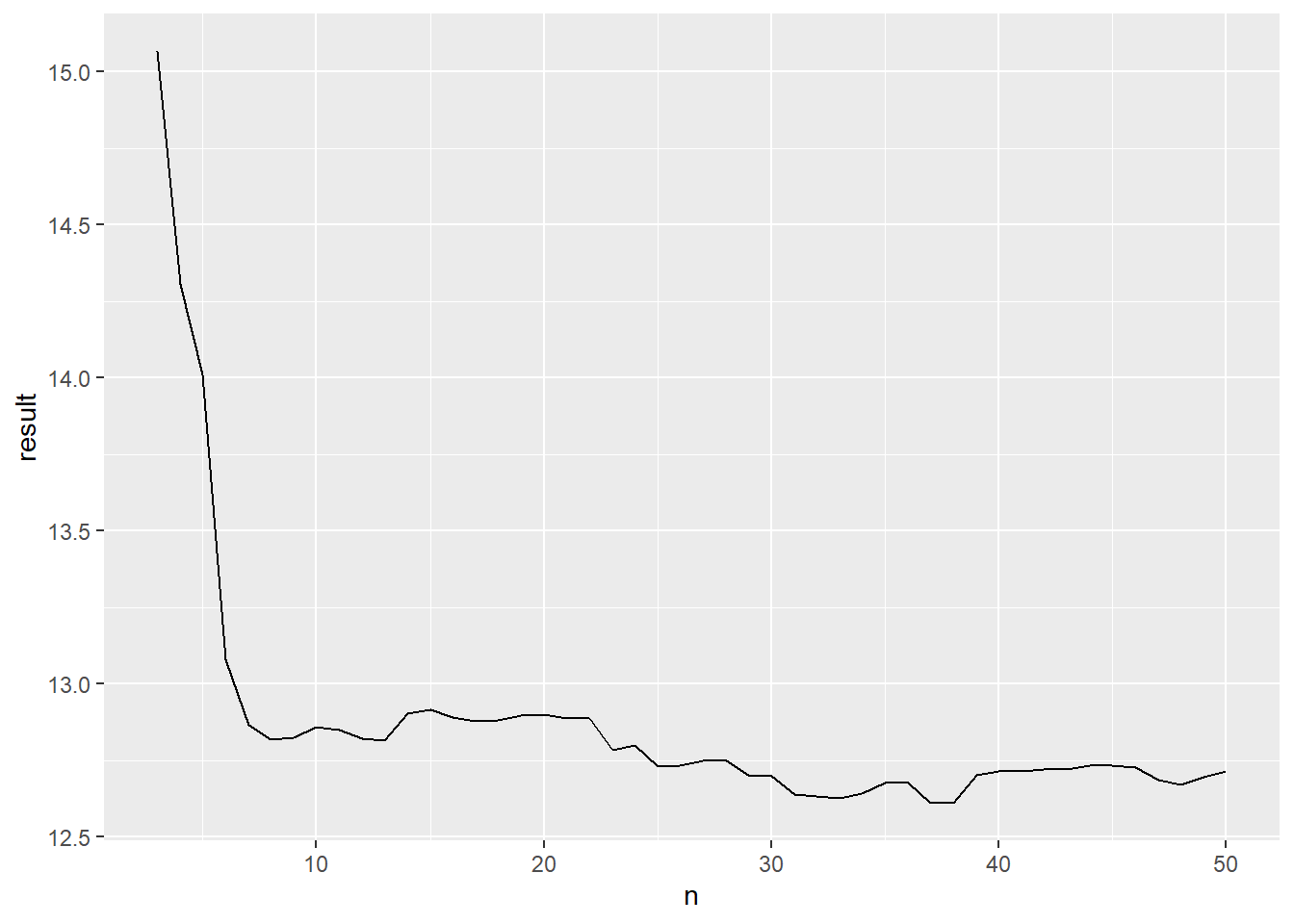least <- min(knn_multiple$result)
best_k <- knn_multiple[knn_multiple\$result == least, 1]

paste("The best k values for KNN model appears to be ", best_k, " with the RMSE of ", least)
##  "The best k values for KNN model appears to be  38  with the RMSE of  12.6103025866823"

## Conclusion

• Running the test multiple times shows that these 3 basic models will give an RMSE of 11 - 13 for math.test score.

• By referring to notebook from another Kaggle’s user, Student Performance Regressor by Joseph Chan, I agree that the parameters provided to this dataset is insufficient to make an accurate prediction on the test score. Furthermore, predicting continuous variable from discrete values isn't a good choice.

• However, by referring to some of the plots I made above, here are some conclusions that I can make:

• Female students perform better in reading and writing while male students perform better in Mathematics, but average are approximately the same.
• Students who ate standard lunch performed better in exam overall.
• Students who took preparation course performed better in exam overall.
• Students of race E appeared to be performing better in exam while students of race A performed slightly worse.
• Students with parents of education level of high school did slightly worse in comparison to other students.